# 深度学习（二十三）Maxout网络学习

Maxout网络学习

本篇博文主要讲解2013年，ICML上的一篇文献：《Maxout  Networks》，这个算法我目前也很少用到，个人感觉最主要的原因应该是这个算法参数个数会成k倍增加(k是maxout的一个参数)，不过没关系，对于我们来说知识积累才是最重要的，指不定某一天我们就需要用到这个算法，技多不压身。个人感觉Maxout网络和Dropout有很多相似的地方。

本篇博文将从什么是maxout网络讲起，先解答maxout的源码层实现，因为很多人最感兴趣的还是算法要怎么实现，当然我也是这样的。我看文献，一般最在意的还是源码的实现，有的文献理论公式推导了十几页，结果5行代码搞定，我看到想哭，这也许就是我讨厌做学术研究的原因吧。知道了源码怎么实现后，我们简单啰嗦一下maxout相关的理论意义。

1、算法概述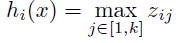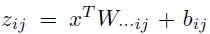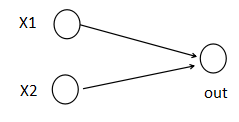(1)以前MLP的方法。我们要计算第i+1层，那个神经元的激活值的时候，传统的MLP计算公式就是：

z=W*X+b

out=f(z)

(2)Maxout 的方法。如果我们设置maxout的参数k=5，maxout层就如下所示：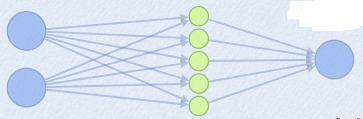z1=w1*x+b1

z2=w2*x+b2

z3=w3*x+b3

z4=w4*x+b4

z5=w5*x+b5

out=max(z1,z2,z3,z4,z5)

ok，为了学习maxout源码的实现过程，我这边引用keras的源码maxout的实现，进行讲解。keras的网站为：http://keras.io/   。项目源码网站为：https://github.com/fchollet/keras。下面是keras关于maxout网络层的实现函数：

#maxout 网络层类的定义
class MaxoutDense(Layer):
# 网络输入数据矩阵大小为(nb_samples, input_dim)
# 网络输出数据矩阵大小为(nb_samples, output_dim)
input_ndim = 2
#nb_feature就是我们前面说的k的个数了，这个是maxout层特有的参数
def __init__(self, output_dim, nb_feature=4,
init='glorot_uniform', weights=None,
W_regularizer=None, b_regularizer=None, activity_regularizer=None,
W_constraint=None, b_constraint=None, input_dim=None, **kwargs):
self.output_dim = output_dim
self.nb_feature = nb_feature
self.init = initializations.get(init)

self.W_regularizer = regularizers.get(W_regularizer)
self.b_regularizer = regularizers.get(b_regularizer)
self.activity_regularizer = regularizers.get(activity_regularizer)

self.W_constraint = constraints.get(W_constraint)
self.b_constraint = constraints.get(b_constraint)
self.constraints = [self.W_constraint, self.b_constraint]

self.initial_weights = weights
self.input_dim = input_dim
if self.input_dim:
kwargs['input_shape'] = (self.input_dim,)
self.input = K.placeholder(ndim=2)
super(MaxoutDense, self).__init__(**kwargs)
#参数初始化部分
def build(self):
input_dim = self.input_shape

self.W = self.init((self.nb_feature, input_dim, self.output_dim))#nb_feature是我们上面说的k。
self.b = K.zeros((self.nb_feature, self.output_dim))

self.params = [self.W, self.b]
self.regularizers = []

if self.W_regularizer:
self.W_regularizer.set_param(self.W)
self.regularizers.append(self.W_regularizer)

if self.b_regularizer:
self.b_regularizer.set_param(self.b)
self.regularizers.append(self.b_regularizer)

if self.activity_regularizer:
self.activity_regularizer.set_layer(self)
self.regularizers.append(self.activity_regularizer)

if self.initial_weights is not None:
self.set_weights(self.initial_weights)
del self.initial_weights

def get_output(self, train=False):
X = self.get_input(train)#需要切记这个x的大小是(nsamples,input_num)
# -- don't need activation since it's just linear.
output = K.max(K.dot(X, self.W) + self.b, axis=1)#maxout激活函数
return output

output = K.max(K.dot(X, self.W) + self.b, axis=1)#maxout激活函数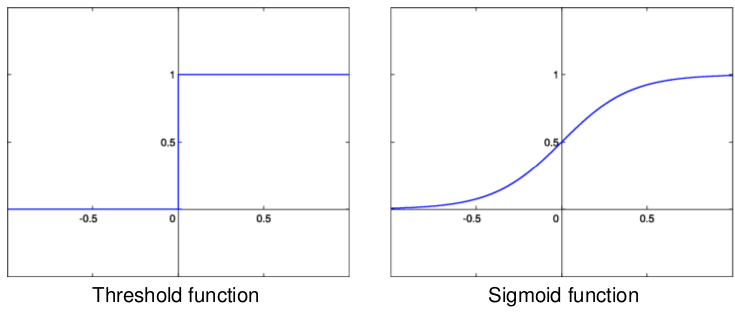1、maxout激活函数并不是一个固定的函数，不像Sigmod、Relu、Tanh等函数，是一个固定的函数方程

2、它是一个可学习的激活函数，因为我们W参数是学习变化的。

3、它是一个分段线性函数：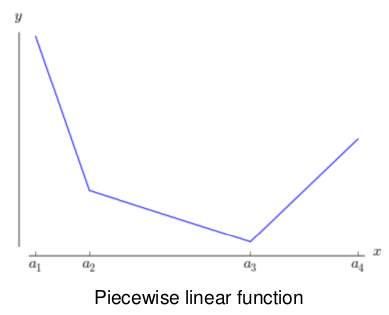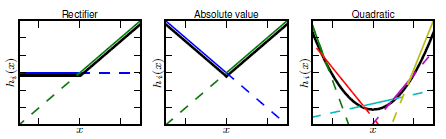maxout是一个函数逼近器对于一个标准的MLP网络来说，如果隐藏层的神经元足够多，那么理论上我们是可以逼近任意的函数的。类似的，对于maxout 网络也是一个函数逼近器。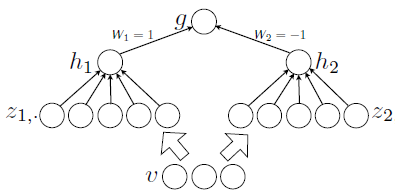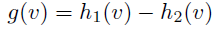1、《Maxout  Networks》

**********************作者：hjimce   时间：2015.12.20  联系QQ：1393852684  原创文章，转载请保留原文地址、作者等信息***************

03-183万+08-241018
08-114669
12-276万+
03-1222万+
02-021万+
08-221万+
10-286万+
02-294128
10-031518